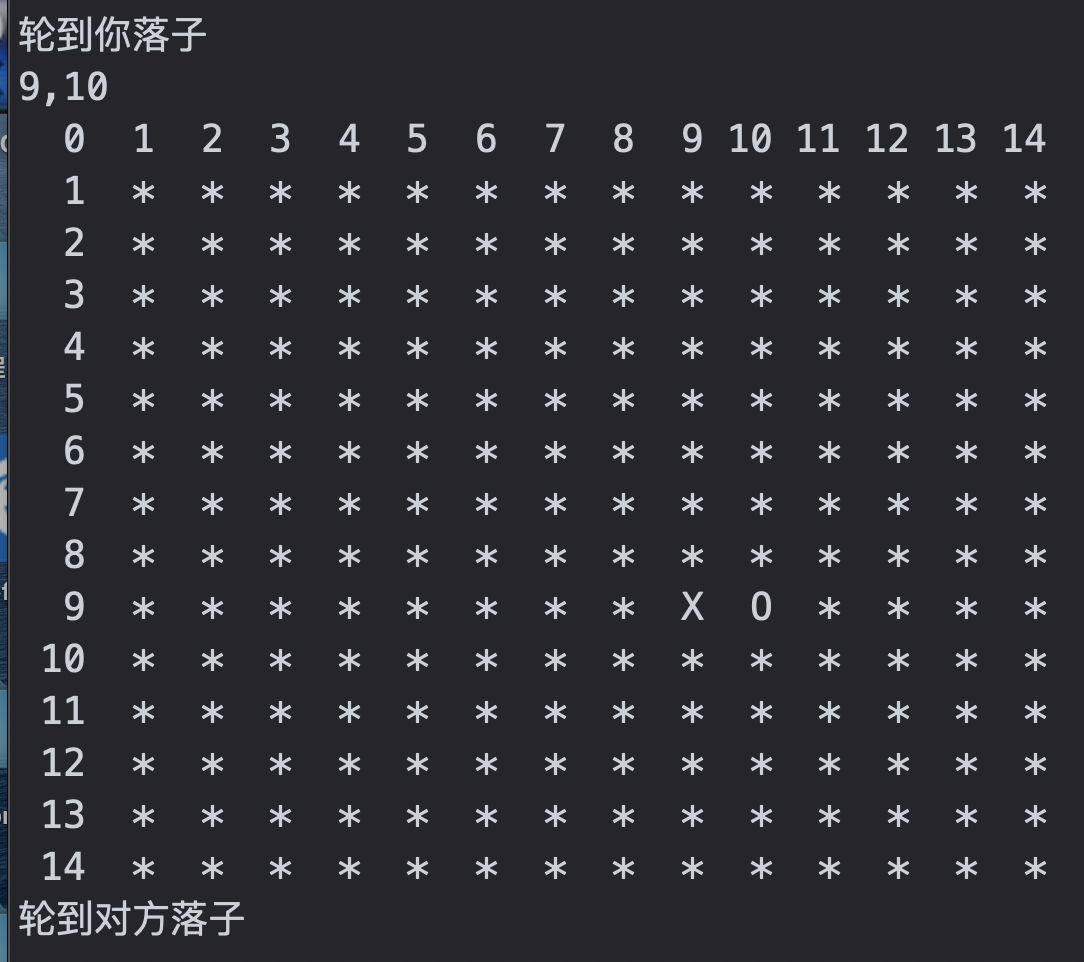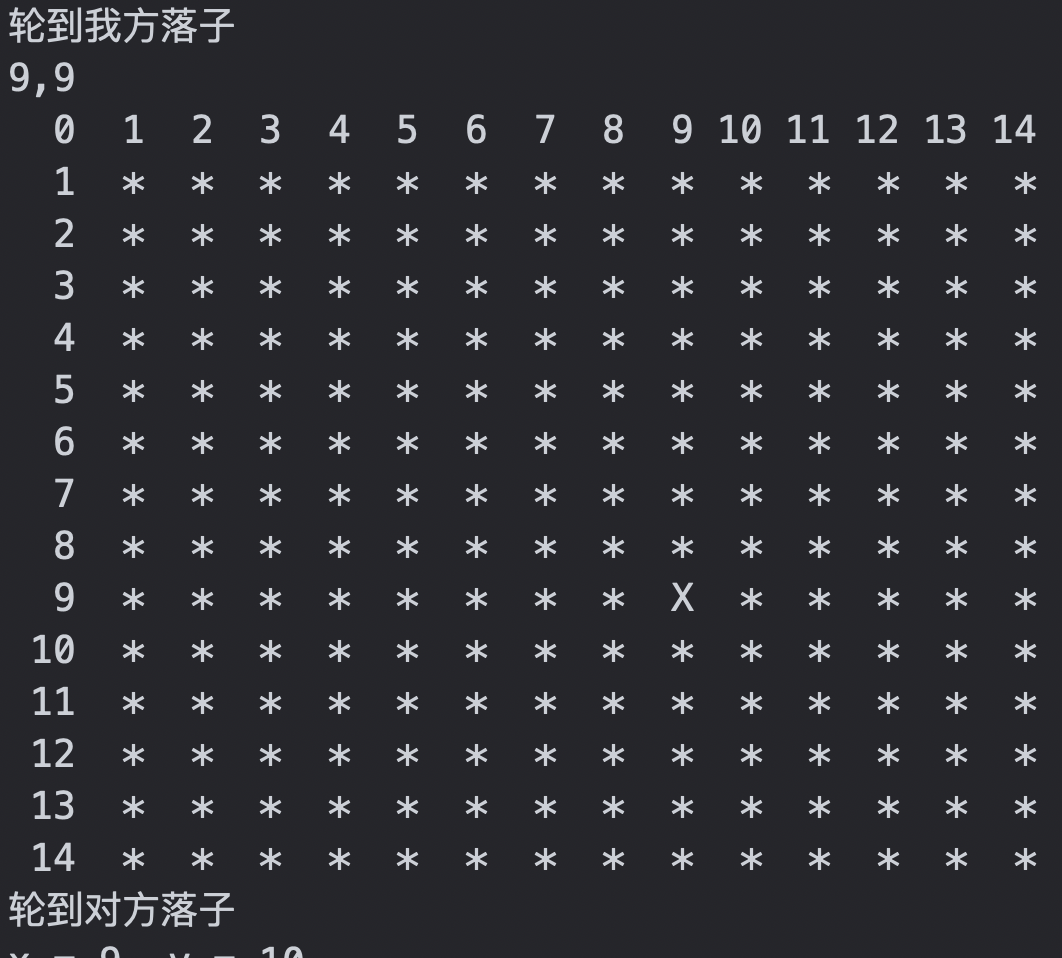• 原标题：C语言制作简单的五子棋游戏 C语言制作简单的五子棋游戏学习C语言的人很多，但是用C语言很少，而用来为自己所用，来做游戏的人就更少了，很多人都是跟着学校学习，学校讲到哪就坐到哪，但是以后却还是不会做...

原标题：C语言制作简单的五子棋游戏C语言制作简单的五子棋游戏
学习C语言的人很多，但是用C语言很少，而用来为自己所用，来做游戏的人就更少了，很多人都是跟着学校学习，学校讲到哪就坐到哪，但是以后却还是不会做项目。今天我就来写一段，如何用C语言来写一个五子棋游戏。(PS：代码有点长，所以前面的字体以及框架怎么写，我就不写下来了，主要是写游戏操作设置以及游戏界面。)代码如下：
#include //图形库
#include //C语言std头文件
#include //kbhit()//按键响应
#include
#pragma comment(lib,"winmm.lib")
//玩游戏
void PlayGame()
{
//鼠标操作
int chess[N][N] = { 0 };//标志没有棋子的标志
while (true)
{
//矫正鼠标坐标
int x, y,i,j;
MOUSEMSG m;
m = GetMouseMsg();
switch (m.uMsg)
{
case WM_LBUTTONDOWN:
//鼠标左键按下画器
if (m.x >= 10 && m.x <= 530 && m.y >= 10 && m.y <= 530)
{
x = m.x % 20;
if (x <= 10)
m.x = m.x - x;
else
m.x = m.x - x + 20;
y = m.y % 20;
if (y <= 10)
m.y = m.y - y;
else
m.y = m.y - y + 20;
i = m.x / 20;
j = m.y / 20;
if (chess[i][j] != 0)
continue;
setlinecolor(BLACK);
setfillcolor(BLACK);
fillcircle(m.x + 10, m.y - 10, 8);
chess[i][j] = 1; //有黑棋1
if (GameOver1(chess, 1) == 1)
{
settextcolor(RED);
settextstyle(50, 50, L"锐字云字库行楷体1.0");
outtextxy(200, 200, L"此局黑棋胜出");
}
break;
}
case WM_RBUTTONDOWN:
if (m.x >= 10 && m.x <= 530 && m.y >= 10 && m.y <= 530)
{
x = m.x % 20;
if (x <= 10)
m.x = m.x - x;
else
m.x = m.x - x + 20;
y = m.y % 20;
if (y <= 10)
m.y = m.y - y;
else
m.y = m.y - y + 20;
i = m.x / 20;
j = m.y / 20;
if (chess[i][j] != 0)
continue;
setlinecolor(WHITE);
setfillcolor(WHITE);
fillcircle(m.x + 10, m.y - 10, 8);
chess[i][j] = 2;
if (GameOver1(chess, 2) == 1)
{
settextcolor(WHITE);
settextstyle(50, 50, L"锐字云字库行楷体1.0");
outtextxy(200, 200, L"此局白棋胜出");
}
break;
}
}
}
}
//游戏界面
void GameBackground()
{
IMAGE GameBackgroundImage;
int width = GameBackgroundImage.getwidth();
int height = GameBackgroundImage.getheight();
initgraph(width, height);
putimage(0, 0, &GameBackgroundImage);
//画棋盘
setlinecolor(BLACK);
for (int i = 10; i <= 530; i += 20)
{
//x,y x1 ,y1
line(10, i, 530, i);
line(i, 10, i, 530);
}
//调整棋盘
//调整边框---加粗
setlinestyle(PS_SOLID, 4);
line(10, 10, 10, 530); //上边加粗
line(10, 530, 530, 530);//下边加粗
line(10, 10, 530, 10);//左边
line(530, 10, 530, 530);//右边
//画点 圆心坐标 +半径
//中间
fillcircle(270, 270, 2);
//左上270-9*20
fillcircle(90, 270, 2);
fillcircle(270, 90, 2);
//右下角 270+9*20
fillcircle(450, 90, 2);
fillcircle(90, 450, 2);
fillcircle(450, 450, 2);
fillcircle(90, 90, 2);
fillcircle(450, 270, 2);
fillcircle(270, 450, 2);
//提示框
messageBox();
PlayGame();
}
int main()
{
GameBegin();
GameBackground();
closegraph();
return 0;
}
代码就是这么多，但是不要直接就复制粘贴咯，因为这串代码是没有前面些窗口的代码的，这个需要你们自己去写，代码只能给你们一些学习的思路，希望你们能学到些东西。
想成为一个合格的C/C++程序员，需要学习哪些内容
首先，学习C/C++肯定要学习C语言(当然，不学习C语言直接学习C++也可以，但是学习C语言把基础打好对以后学习其他编程语言都是会有帮助的)
今天就先来讲讲从基础到进阶，C语言基础该怎么学习
——初级阶段，对于完全不懂的小白来说，首先需要了解计算机的组成原理(当然在如今这个时代，一般的都是知道的)然后开始学习理论，编程的启蒙思维(当然这前两个都是了解下就差不多了，不用太着重去学习)然后就需要开始学习内容了——首先先学习一些基本的数据类型，然后还有运算符，顺序结构，分支结构，循环结构，当你已经能运用这些基础的知识内容写一个小程序，恭喜你，你开始入门了，但是仅仅只是个开始。。。
——现在进入中级阶段，学习的内容会比较多，需要学习数组，函数，指针(指针方面包含很多内容，这方面需要着重的学习，对后续的学习方面帮助会很大)，预处理指令，结构体与公用体，枚举，位运算，联合。。这些是中级阶段的学习内容，虽然打出来显得很少，但是接触的内容会很多，大家要多多着重学习(加群：594089997，学习C/C++)
——最后就是进入高级学习阶段了，学习的分类比较少，但是每个分类里面包含的内容确实最难的，千万不要因为文字打出来这么简单就懈怠咯。。高级部分包含的内容主要就是数据结构，动态储存管理，高级图形编程，Socket通信编程，其中数据结构包含了数据结构--线性表，数据结构--栈和队列，数据结构--串，数据结构--常用算法，这些内容对你们以后往C++方面或者是嵌入式方面发展都有着不可小觑的作用
关注微信公众号：编程语言
如果对这方面感兴趣或者在学习C/C++的过程中有什么问题的话，可以加群：594089997，群内有大量C/C++资料，也会有人解答问题，大家一起学习，一起交流。希望每位学习C/C++的人都能学有所成。
有更多(C/C++语言，linux，JAVA语言)高手帮助你解决难题，一起互动，提高大家的编程水平返回搜狐，查看更多
责任编辑：

展开全文• 这是本人在备考复试的时候结合之前自己写的一个五子棋游戏，以及学习计算机网络时写的套接字编程而写的联机版五子棋游戏。由于时间原因，还有很多小细节没有来得及实现，不严格按照输入来会出现bug，以后要是有时间...
这是本人在备考复试的时候结合之前自己写的一个五子棋游戏，以及学习计算机网络时写的套接字编程而写的联机版五子棋游戏。由于时间原因，还有很多小细节没有来得及实现，不严格按照输入来会出现bug，以后要是有时间会考虑进行完善。 在此也推荐下初学计算机的人可以看看计算机网络自顶向下，写的十分棒的一本书，同时可以结合MOOC哈工大的计算机网络学习效果贼棒！！！ 以下为后手（服务器端）代码
#include <stdio.h>
#include <sys/socket.h>
#include <stdlib.h>
#include <netinet/in.h>
#include <string.h>
#include <unistd.h>
#include <arpa/inet.h>

#define N 15
struct locate{
int x;
int y;
};
int map[N][N];

void printchessmap(){
for(int i=0;i<N;i++){
for(int j=0;j<N;j++){
if(i==0){
printf("%3d",j);
}
else if(j==0){
printf("%3d ",i);
}
else if(1 == map[i][j]){
printf(" X ");
}
else if(2 == map[i][j]){
printf(" O ");
}
else{
printf(" * ");
}
}
printf("\n");
}
}

int judge(int x,int y){
int w=1,k=1,l=1,r=1;
for(int i=1;i<5;i++){               //w判断竖向
if(map[x+i][y]==map[x][y]&&x+i<N)
w++;
else
break;
}
for(int i=1;i<5;i++){
if(map[x-i][y]==map[x][y]&&x-i>0)
w++;
else
break;
}
if(w>=5)
return 1;
for(int i=1;i<5;i++){
if(map[x][y+i]==map[x][y]&&y+i<N)
k++;
else
break;
}
for(int i=1;i<5;i++){
if(map[x][y-i]==map[x][y]&&y-i>0)
k++;
else
break;
}
if(k>=5)
return 1;
for(int i=1;i<5;i++){
if(map[x+i][y+i]==map[x][y]&&x+i<N&&y+i<N)
l++;
else
break;
}
for(int i=1;i<5;i++){
if(map[x-i][y-i]==map[x][y]&&x-i>0&&y-i>0)
l++;
else
break;
}
if(l>=5)
return 1;
for(int i=1;i<5;i++){
if(map[x+i][y-i]==map[x][y]&&x+i<N&&y-i>0)
r++;
else
break;
}
for(int i=1;i<5;i++){
if(map[x-i][y+i]==map[x][y]&&x-i>0&&y+i>0)
r++;
else
break;
}
if(r>=5)
return 1;

return 0;
}

int main(int argc, char *argv[]) {
/*创建socket套接字*/
int _sock = socket(AF_INET, SOCK_STREAM, IPPROTO_TCP); //定义族、socket类型、协议类型
if(_sock==-1){
printf("socket build error!\n");//创建失败返回错误
return 1;
}else{
printf("socket build success\n");
}
/*绑定端口地址*/
printf("bind error!\n");
return 1;
}else{
printf("bind success\n");
}
/*监听*/
if(listen(_sock,5)==-1){		//套接字最大允许连接数量
printf("listen error!\n");
}else{
printf("listen success\n");
}
/*等待客户端连接accept*/
if(_cSock == -1){
perror("client socket error");
}else{
}
/*********五子棋准备*********/
for(int i=0;i<N;i++){             //初始化
for(int j=0;j<N;j++){
map[i][j]=0;
}
}
printchessmap();                 //打印棋盘
printf("等待对方输入\n");
/**************************/
/*循环接受数据*/
struct locate senddp;
while(1){
char recvBuf;
int nLen = recv(_cSock, recvBuf,256, 0);                 //接收客户端数据
if(nLen <= 0){
printf("client has quit!\n");
break;
}
struct locate *clocate = (struct locate*)recvBuf;
printf("x = %d, y = %d\n",clocate->x,clocate->y);   //打印客户端数据
map[clocate->x][clocate->y] = 1;  //客户端是1
printchessmap();
int win=judge(clocate->x, clocate->y);
if(win == 1){
printf("客户端胜利！\n");
break;
}else{
printf("轮到你落子\n");
}
int x,y;
scanf("%d,%d",&x,&y);                                   //输入发给客户端数据
senddp.x = x;
senddp.y = y;
send(_cSock, (const char*)&senddp, sizeof(struct locate), 0);  //发送
map[x][y] = 2;
printchessmap();
win= judge(x,y);
if(win == 1){
printf("服务端胜利！\n");
break;
}else{
printf("轮到对方落子\n");
}

}
close(_sock);
return 0;
}

服务器端先启动监听由客户端发来的请求 以下是先手客户端部分代码
#include <stdio.h>
#include <stdlib.h>
#include <netinet/in.h>
#include <sys/socket.h>
#include <arpa/inet.h>
#include <unistd.h>

#define SOCKET_ERROR -1
#define N 15

int map[N][N];
struct DataPackage{
int x;
int y;
};

void printchessmap(){
for(int i=0;i<N;i++){
for(int j=0;j<N;j++){
if(i==0){
printf("%3d",j);
}
else if(j==0){
printf("%3d ",i);
}
else if(1 == map[i][j]){
printf(" X ");
}
else if(2 == map[i][j]){
printf(" O ");
}
else{
printf(" * ");
}
}
printf("\n");
}
}

int judge(int x,int y){
int w=1,k=1,l=1,r=1;
for(int i=1;i<5;i++){               //w判断竖向
if(map[x+i][y]==map[x][y]&&x+i<N)
w++;
else
break;
}
for(int i=1;i<5;i++){
if(map[x-i][y]==map[x][y]&&x-i>0)
w++;
else
break;
}
if(w>=5)
return 1;
for(int i=1;i<5;i++){ 				//k横向
if(map[x][y+i]==map[x][y]&&y+i<N)
k++;
else
break;
}
for(int i=1;i<5;i++){
if(map[x][y-i]==map[x][y]&&y-i>0)
k++;
else
break;
}
if(k>=5)
return 1;
for(int i=1;i<5;i++){				//l正斜向
if(map[x+i][y+i]==map[x][y]&&x+i<N&&y+i<N)
l++;
else
break;
}
for(int i=1;i<5;i++){
if(map[x-i][y-i]==map[x][y]&&x-i>0&&y-i>0)
l++;
else
break;
}
if(l>=5)
return 1;
for(int i=1;i<5;i++){				//r反斜向
if(map[x+i][y-i]==map[x][y]&&x+i<N&&y-i>0)
r++;
else
break;
}
for(int i=1;i<5;i++){
if(map[x-i][y+i]==map[x][y]&&x-i>0&&y+i>0)
r++;
else
break;
}
if(r>=5)
return 1;

return 0;
}

int main() {
/*建立socket*/
int _sock = socket(AF_INET, SOCK_STREAM, 0);//与服务器端不同第三个参数无需声明
if(_sock == SOCKET_ERROR){
printf("socket build error \n");
}
else{
printf("socket build success \n");
}

/*连接服务器*/
_sin.sin_family = AF_INET;
_sin.sin_port = htons(1997);
if(ret == SOCKET_ERROR){
printf("connect error \n");
}
else{
printf("connect success \n");
}
/*五子棋准备*/
for(int i=0;i<N;i++){			//初始化棋盘
for(int j=0;j<N;j++){
map[i][j]=0;
}
}
printchessmap();
/*接收服务器信息*/
char recvBuf = {};
while(1){
printf("轮到我方落子\n");
int x,y;
scanf("%d,%d",&x,&y);
map[x][y] = 1;
struct DataPackage senddp;
senddp.x = x;
senddp.y = y;
printchessmap();
int win = judge(x, y);
send(_sock, (const char*)&senddp, sizeof(struct DataPackage), 0);    //发送数据给服务端
if(win == 1){
printf("你赢了！\n");
break;
}
printf("轮到对方落子\n");
char recvBuf;
int nLen = recv(_sock, recvBuf,256, 0);                              //接收数据
if(nLen <= 0){
printf("server has quit!\n");
break;
}
struct DataPackage *clocate = (struct DataPackage*)recvBuf;
printf("x = %d, y = %d\n",clocate->x,clocate->y);	//打印数据
map[clocate->x][clocate->y] = 2;
printchessmap();
win = judge(clocate->x, clocate->y);
if(win == 1){
printf("对方胜利!\n");
break;
}

}
close(_sock);
return 0;
}

出来的效果如图展开全文• 该楼层疑似违规已被系统折叠隐藏此楼查看此楼return true;}x++;}return false;}bool isLeftInclinedWin(bool isBlack,int x,int y){char c = isBlack ? '@':'O';int count;while(x>0 &&...

该楼层疑似违规已被系统折叠 隐藏此楼查看此楼
return true;
}
x++;
}
return false;
}
bool isLeftInclinedWin(bool isBlack,int x,int y)
{
char c = isBlack ? '@':'O';
int count;
while(x>0 && y>0 && state[x][y] == c)
{
y--;
x--;
}
count =0;
if(state[x][y] == c) count = 1;
x++;
y++;
while(x < N && y < N && state[x][y] == c)
{
count++;
if(count == 5)
{
return true;
}
x++;
y++;
}
return false;
}
bool isRightObliqueWin(bool isBlack,int x,int y)
{
char c = isBlack ? '@':'O';
int count;
while(x>0 && y
{
y++;
x--;
}
count =0;
if(state[x][y] == c) count = 1;
x++;
y--;
while(x < N && y >= 0 && state[x][y] == c)
{
count++;
if(count == 5)
{
return true;
}
x++;
y--;
}
return false;
}
void init(void)
{
int i,j;
for(i=0;i
{
for(j=0;j
{
state[i][j] = '*';

展开全文• 五子棋游戏源代码，C语言实现，是我从程序员网上下的，在此分享一下
• #pragma comment(lib,'winmm.lib')//玩游戏void PlayGame(){//鼠标操作int chess[N][N] = { 0 };//标志没有棋子的标志while (true){//矫正鼠标坐标int x, y,i,j;MOUSEMSG m;m = GetMouseMsg();switch (m.uMsg){case ...

#pragma comment(lib,'winmm.lib')
//玩游戏
void PlayGame()
{
//鼠标操作
int chess[N][N] = { 0 };//标志没有棋子的标志
while (true)
{
//矫正鼠标坐标
int x, y,i,j;
MOUSEMSG m;
m = GetMouseMsg();
switch (m.uMsg)
{
case WM_LBUTTONDOWN:
//鼠标左键按下画器
if (m.x >= 10 && m.x <= 530="" &&="" m.y="">= 10 && m.y <=>=>
{
x = m.x % 20;
if (x <=>=>
m.x = m.x - x;
else
m.x = m.x - x + 20;
y = m.y % 20;
if (y <=>=>
m.y = m.y - y;
else
m.y = m.y - y + 20;
i = m.x / 20;
j = m.y / 20;
if (chess[i][j] != 0)
continue;
setlinecolor(BLACK);
setfillcolor(BLACK);
fillcircle(m.x + 10, m.y - 10, 8);
chess[i][j] = 1; //有黑棋1
if (GameOver1(chess, 1) == 1)
{
settextcolor(RED);
settextstyle(50, 50, L'锐字云字库行楷体1.0');
outtextxy(200, 200, L'此局黑棋胜出');
}
break;
}
case WM_RBUTTONDOWN:
if (m.x >= 10 && m.x <= 530="" &&="" m.y="">= 10 && m.y <=>=>
{
x = m.x % 20;
if (x <=>=>
m.x = m.x - x;
else
m.x = m.x - x + 20;
y = m.y % 20;
if (y <=>=>
m.y = m.y - y;
else
m.y = m.y - y + 20;
i = m.x / 20;
j = m.y / 20;
if (chess[i][j] != 0)
continue;
setlinecolor(WHITE);
setfillcolor(WHITE);
fillcircle(m.x + 10, m.y - 10, 8);
chess[i][j] = 2;
if (GameOver1(chess, 2) == 1)
{
settextcolor(WHITE);
settextstyle(50, 50, L'锐字云字库行楷体1.0');
outtextxy(200, 200, L'此局白棋胜出');
}
break;
}
}
}
}
//游戏界面
void GameBackground()
{
IMAGE GameBackgroundImage;
int width = GameBackgroundImage.getwidth();
int height = GameBackgroundImage.getheight();
initgraph(width, height);
putimage(0, 0, &GameBackgroundImage);
//画棋盘
setlinecolor(BLACK);
for (int i = 10; i <= 530;="" i="" +="">=>
{
//x,y x1 ,y1
line(10, i, 530, i);
line(i, 10, i, 530);
}
//调整棋盘
//调整边框---加粗
setlinestyle(PS_SOLID, 4);
line(10, 10, 10, 530); //上边加粗
line(10, 530, 530, 530);//下边加粗
line(10, 10, 530, 10);//左边
line(530, 10, 530, 530);//右边
//画点 圆心坐标 +半径
//中间
fillcircle(270, 270, 2);
//左上270-9*20
fillcircle(90, 270, 2);
fillcircle(270, 90, 2);
//右下角 270+9*20
fillcircle(450, 90, 2);
fillcircle(90, 450, 2);
fillcircle(450, 450, 2);
fillcircle(90, 90, 2);
fillcircle(450, 270, 2);
fillcircle(270, 450, 2);
//提示框
messageBox();
PlayGame();
}
int main()
{
GameBegin();
GameBackground();
closegraph();
return 0;
}
代码就是这么多，但是不要直接就复制粘贴咯，因为这串代码是没有前面些窗口的代码的，这个需要你们自己去写，代码只能给你们一些学习的思路，希望你们能学到些东西。
想成为一个合格的C/C++程序员，需要学习哪些内容
首先，学习C/C++肯定要学习C语言(当然，不学习C语言直接学习C++也可以，但是学习C语言把基础打好对以后学习其他编程语言都是会有帮助的)
今天就先来讲讲从基础到进阶，C语言基础该怎么学习
——初级阶段，对于完全不懂的小白来说，首先需要了解计算机的组成原理(当然在如今这个时代，一般的都是知道的)然后开始学习理论，编程的启蒙思维(当然这前两个都是了解下就差不多了，不用太着重去学习)然后就需要开始学习内容了——首先先学习一些基本的数据类型，然后还有运算符，顺序结构，分支结构，循环结构，当你已经能运用这些基础的知识内容写一个小程序，恭喜你，你开始入门了，但是仅仅只是个开始。。。
——现在进入中级阶段，学习的内容会比较多，需要学习数组，函数，指针(指针方面包含很多内容，这方面需要着重的学习，对后续的学习方面帮助会很大)，预处理指令，结构体与公用体，枚举，位运算，联合。。这些是中级阶段的学习内容，虽然打出来显得很少，但是接触的内容会很多，大家要多多着重学习(加群：594089997，学习C/C++)
——最后就是进入高级学习阶段了，学习的分类比较少，但是每个分类里面包含的内容确实最难的，千万不要因为文字打出来这么简单就懈怠咯。。高级部分包含的内容主要就是数据结构，动态储存管理，高级图形编程，Socket通信编程，其中数据结构包含了数据结构--线性表，数据结构--栈和队列，数据结构--串，数据结构--常用算法，这些内容对你们以后往C++方面或者是嵌入式方面发展都有着不可小觑的作用
=>=>

展开全文• = max){max = cur;p.x = i;p.y = j;p.z = 1;}}}return p;}//鏌ョ湅鍧愭爣x y鏄惁鑳借蛋int if_done(int x,int y){if(x>=CHESSBOARD1||x<0)return 0;if(y>=CHESSBOARD2||y<0)return 0;...
• C语言写的五子棋游戏代码用C语言写的五子棋游戏代码用C语言写的五子棋游戏代码用C语言写的五子棋游戏代码用C语言写的五子棋游戏代码用C语言写的五子棋游戏代码用C语言写的五子棋游戏代码用C语言写的五子棋游戏代码...算法
• 该楼层疑似违规已被系统折叠隐藏此楼查看此楼return true;}x++;}return false;}bool isLeftInclinedWin(bool isBlack,int x,int y){char c = isBlack ? '@':'O';int count;while(x>0 &&...
• 该楼层疑似违规已被系统折叠隐藏此楼查看此楼return true;}x++;}return false;}bool isLeftInclinedWin(bool isBlack,int x,int y){char c = isBlack ? '@':'O';int count;while(x>0 &&...
• 该楼层疑似违规已被系统折叠隐藏此楼查看此楼return true;}x++;}return false;}bool isLeftInclinedWin(bool isBlack,int x,int y){char c = isBlack ? '@':'O';int count;while(x>0 &&...
• 该楼层疑似违规已被系统折叠隐藏此楼查看此楼return true;}x++;}return false;}bool isLeftInclinedWin(bool isBlack,int x,int y){char c = isBlack ? '@':'O';int count;while(x>0 &&...
• ## 双人小游戏—五子棋(c语言)

千次阅读 多人点赞 2021-06-02 11:11:35
实现功能 生成棋盘玩家1与玩家2对战，哪个玩家率先有连续5子连线，哪个玩家赢。 如何实现 组成： 二维数组：board[ROW][COL],定义一个ROW*COL的棋盘。 主要逻辑： ...显示棋盘，提示用户下子，下子后判断， ...
• ## 五子棋代码C语言实现

千次阅读 热门讨论 2020-04-17 22:18:03
五子棋代码C语言实现（数据结构实训） 这是我大一时候组队合作写的五子棋游戏代码，实现了人人对战，人机对战，悔棋，音乐开关功能，当看到界面实现的那一刻，我掉落的头发都是值得的！！！下面贴上源代码（顺便...
• 一个简易的、用C语言写的五子棋游戏源代码，仅供参考！
• 该楼层疑似违规已被系统折叠隐藏此楼查看此楼/*初始化游戏界面：1.绘图环境2.背景图片3.背景音乐4.绘制格子5.绘制边界线6.显示黑棋为玩家1，白棋为玩家2下棋：1.while循环让游戏能不断的玩下去2.定义一个保存鼠标...
• C语言编写的五子棋代码。简单易懂，效率高。就是界面稍微差了点儿
• C语言实现的五子棋游戏 单机版 中文注解 可以规定玩家个数和棋盘大小
• 暑期实训完成的软件，五子棋游戏可以实现人机对战，分了两种难度，还可以人人对战，重点来了，可以联机可以联机可以联机，在两台电脑上对战，就此这个软件获得了我们学院的三等奖，下载绝不后悔
• easyx游戏编程：五子棋C语言游戏开发）源码 源码介绍 我的编程环境是vs2019 #include "stdafx.h"是自带的，其他版本可能需要添加#include “stdafx.h” 除了下面代码外，无其他改动 #include #include #include #......

# 五子棋游戏c语言c语言 订阅